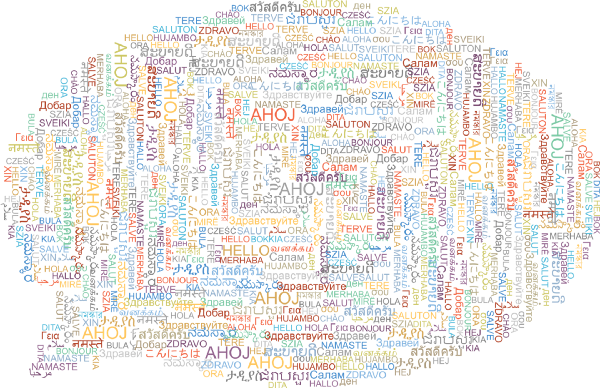Announcements ·December 19, 2018
Posted by Laurence Moroney and Edward LoperTensorFlow now supports Unicode, a standard encoding system used to represent characters from almost all languages. When processing natural language, it can be important to understand how characters are encoded in byte strings. In languages with small character sets like English, each character can be encoded in a single byte using the ASCII encoding. But this approach is not practical for other languages, such as Chinese, which have thousands of characters. Even when processing English text, special characters such as Emojis cannot be encoded with ASCII.

The most common standard for defining characters and their encodings is Unicode, which supports virtually all languages. With Unicode, each character is represented using a unique integer code point with a value between `0` and `0x10FFFF`. When code points are put in sequence, a Unicode string is formed.

The new Unicode tutorial colab shows how to represent Unicode strings in TensorFlow. When using TensorFlow there are two standard ways to represent a Unicode string:
• As a vector of integers, where each position contains a single code point.
• As a string, where the sequence of code points is encoded into the string using a character encoding. There are many character encodings, with some of the most common being UTF-8, UTF-16 and more.
The following code shows encodings for the string `"语言处理"` (which means “language processing” in Chinese) using code points, UTF-8 and UTF-16 respectively.
``````# Unicode string, represented as a vector of code points.
text_chars = tf.constant([35821, 35328, 22788, 29702])

# Unicode string, represented as a UTF-8-encoded string scalar.

# Unicode string, represented as a UTF-16-BE-encoded string scalar.
text_utf16be = tf.constant('\x8b\xed\x8a\x00Y\x04t\x06')``````
Naturally you may need to convert between representations — and TensorFlow 1.13 has added functions to do this:
• `tf.strings.unicode_decode`: Converts an encoded string scalar to a vector of code points.
• `tf.strings.unicode_encode`: Converts a vector of code points to an encoded string scalar.
• `tf.strings.unicode_transcode`: Converts an encoded string scalar to a different encoding.
So, for example, if you want to decode the UTF-8 representation from the above examples into a vector of code points, you would do the following:
``````>>> tf.strings.unicode_decode(text_utf8, input_encoding='UTF-8')
tf.Tensor([35821 35328 22788 29702], shape=(4,), dtype=int32)``````
When decoding a `Tensor` containing multiple strings, the strings may have differing lengths. `unicode_decode` returns the result as a `RaggedTensor`, where the length of the inner dimension varies depending on the number of characters in each string.
``````>>> hello = [b"Hello", b"你好", b"こんにちは", b"Bonjour"]
>>> tf.strings.unicode_decode(hello, input_encoding='UTF-8')
``````
To learn more about Unicode support in TensorFlow, check out the Unicode tutorial colab and browse the `tf.strings` documentation.
Next postPosted by Laurence Moroney and Edward Loper

TensorFlow now supports Unicode, a standard encoding system used to represent characters from almost all languages. When processing natural language, it can be important to understand how characters are encoded in byte strings. In languages with small character sets like English, each character can be encoded in a single byte using the ASCII encoding. B…